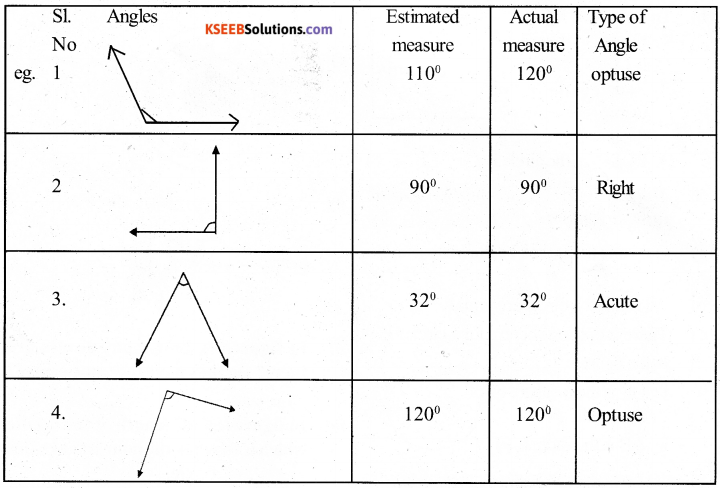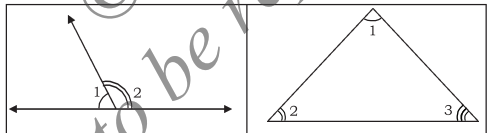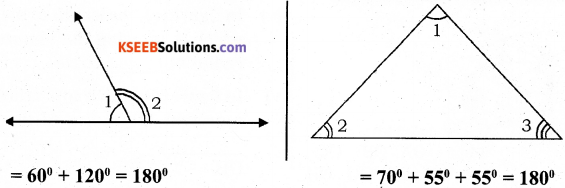# KSEEB Solutions for Class 5 Maths Chapter 6 Angles

Students can Download Maths Chapter 6 Angles Questions and Answers, Summary, Notes Pdf, KSEEB Solutions for Class 5 Maths helps you to revise the complete Karnataka State Board Syllabus and score more marks in your examinations.

## Karnataka State Syllabus Class 5 Maths Chapter 6 Angles

### KSEEB Class 5 Maths Angles Ex 6.1

Question 1.
Observe your surrounding environment and list the situations where the angles are formed.
Room, clock, pillars of a building, roof of a building, water tank, ball etc.Question 2.
Observe the angles formed while doing the following yogasana postures. Certain angles are already marked to help you.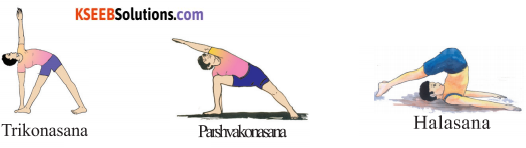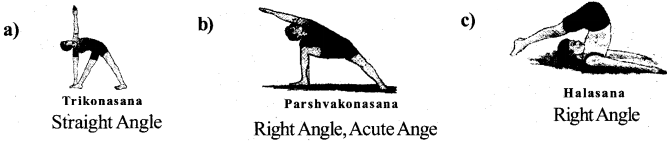Question 3.
Pranav has arranged sticks as shown in the figure. Observe the angles formed here and mark the angles by drawing lines.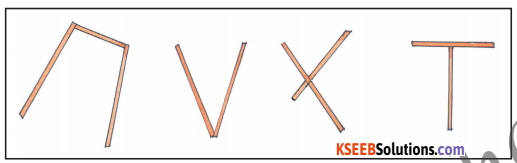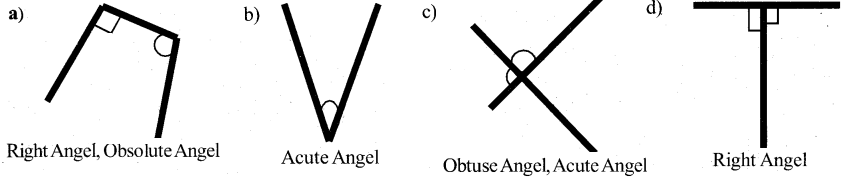Question 4.
Name the angle, vertex and the sides in the following figures.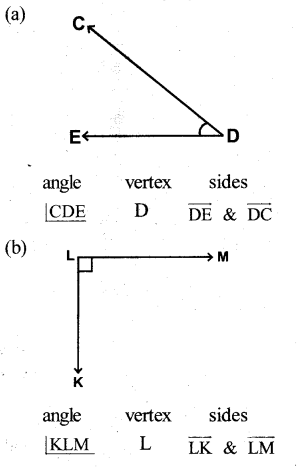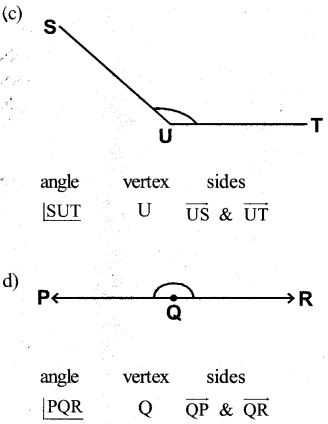### KSEEB Class 5 Maths Angles Ex 6.2

Question 1.
Mark the right angle, acute angle and obtuse angle with different colours in the following figures.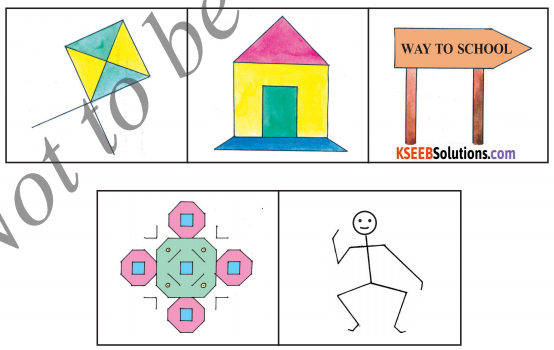a)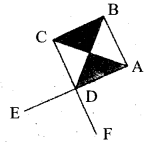RightAngel ∠EDF ∠CDE ∠CBA ∠BCD
∠COB ∠AOD ∠AOB
Acute Angel ∠CBO ∠OCB ∠OBA ∠BAO

b)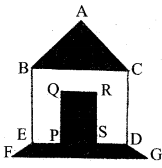RightAngel ∠BAC ∠BEP ∠CDS ∠PQR
∠QRS ∠RSP ∠SPQ ∠EBC ∠BCD
Acute Angel ∠ABC ∠ACB ∠EFG ∠DGF
ObtuseAngle: ∠BEF ∠CDG ∠FED ∠GDE

c)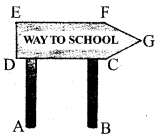Right Angel: ∠DEF ∠EDC ∠ADC ∠BCD
AcuteAngel: ∠FGC
Obtuse Angle: ∠DCG ∠EFG

d)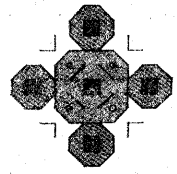Right Angle – Blue colour
Obtuse Angle – Pink colour, Sky blue

e)Right Angle: ∠ABC ∠ABD
Complete Angle: ∠A
ObtuseAngle: ∠BCL ∠CLM ∠HKN ∠IJO ∠PFE
Acute Angel: ∠DEFQuestion 2.
Measure the following angles and write the measurement and type of each angle.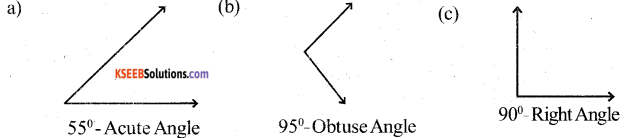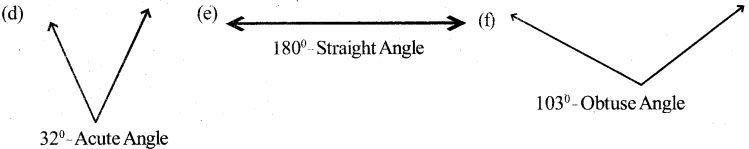Question 3.
Observe the acute angle, obtuse angle and right angles in your classroom and list them.
Two edges of wall of a classroom – Right Angle
Two edges of a door – Right Angle
Two edges of a window – Right AngleQuestion 4.
Write your name using English capital letters. List the number of acute angles, obtuse angles and right angles in them.
Refer to StudentsQuestion 5.
Draw any six angles using scale and measure them using a protractor.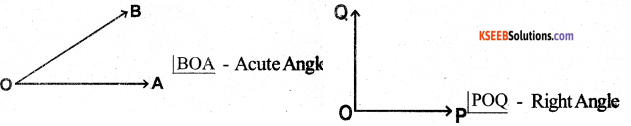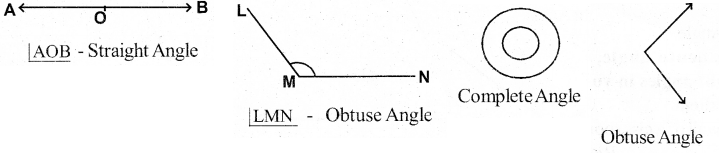Question 6.
Write the measure of the angle between the two hands of the following docks and name them.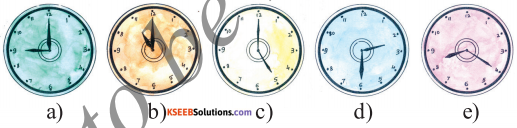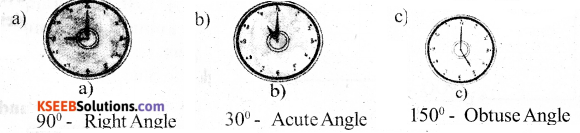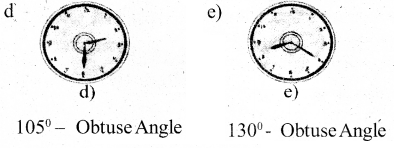Question 7.
Choose the right answer from the following.
a) Example for obtuse angle.
1) 90°
2) 53°
3) 178°
4) 180°
3) 178° – Obtuse Angle

b) In the given figure, the number of right angles, acute angles and obtuse angles are
1) 15, 4, 10
2) 4, 15, 10
3) 10, 10, 4
4) 4, 5, 5
2) 4, 15,10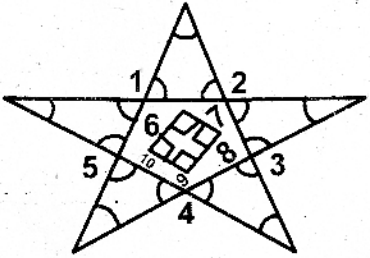Question 8.
Classify the measures of angles given below :
16°, 180°, 88°, 179°, 45°, 90°, 100°, 35°, 142°.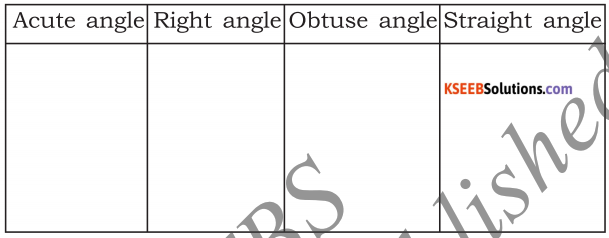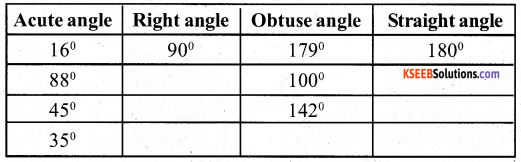Question 9.
Estimate the measure of the following angles.
Verify by measuring them. Name the type of angles.